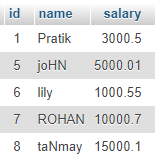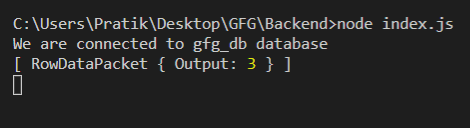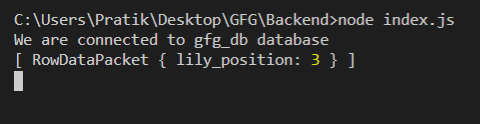GeeksforGeeks App
Open AppBrowser
Continue

# Node.js MySQL FIND_IN_SET() Function

FIND_IN_SET() function is a built-in function in MySQL that is used to get the position of the first occurrence of value string in a list of strings separated by comma(‘,’).

Syntax:

`FIND_IN_SET(value, list_of_string)`

Parameters: It takes two parameters as follows:

• value: It is the value to be searched.
• list_of_string: It is the list of strings separated by comma (‘,’).

Return Value: It returns the position of first occurrence of value string in a list of string separated by comma (‘,’)

Module Installation: Install the mysql module using the following command:

`npm install mysql`

Database: Our SQL publishers table preview with sample data is shown below:Example 1:

## index.js

 `const mysql = require(``"mysql"``);`` ` `let db_con  = mysql.createConnection({``    ``host: ``"localhost"``,``    ``user: ``"root"``,``    ``password: ``''``,``    ``database: ``'gfg_db'``});`` ` `db_con.connect((err) => {``    ``if` `(err) {``      ``console.log(``"Database Connection Failed !!!"``, err);``      ``return``;``    ``}`` ` `    ``console.log(``"We are connected to gfg_db database"``);`` ` `    ``// Here is the query``    ``let query = ``"SELECT FIND_IN_SET('2', '1,12,2,32') AS Output"``;`` ` `    ``db_con.query(query, (err, rows) => {``        ``if``(err) ``throw` `err;`` ` `        ``console.log(rows);``    ``});``});`

Run the index.js file using the following command:

`node index.js`

Output:## index.js

 `const mysql = require(``"mysql"``);`` ` `let db_con  = mysql.createConnection({``    ``host: ``"localhost"``,``    ``user: ``"root"``,``    ``password: ``''``,``    ``database: ``'gfg_db'``});`` ` `db_con.connect((err) => {``    ``if` `(err) {``      ``console.log(``"Database Connection Failed !!!"``, err);``      ``return``;``    ``}`` ` `    ``console.log(``"We are connected to gfg_db database"``);`` ` `    ``// Here is the query``    ``let sub_query = ``"(SELECT GROUP_CONCAT(name) FROM publishers)"``;`` ` `    ``let main_query = ```SELECT FIND_IN_SET(``'lily'``, \${sub_query}) AS lily_position`;`` ` `    ``db_con.query(main_query, (err, rows) => {``        ``if``(err) ``throw` `err;`` ` `        ``console.log(rows);``    ``});``});`

Run the index.js file using the following command:

`node index.js`

Output:My Personal Notes arrow_drop_up
Related Tutorials